When determining the structure of a compound or detecting what compounds are present, one of the best methods is mass spectrometry (MS).

When measuring a known compound, mass spectrometry can determine if it is the target compound with a high probability. Even if it is an unknown compound, mass spectrometry can be used to measure molecular weight. From this, we can infer the structure of the molecule.

In both organic chemistry and biochemistry, MS is an analytical instrument that is used by a great many researchers. Therefore, it is necessary to understand how to utilize mass spectrometry.

Aside from understanding the principles of MS, you also need to learn how to read spectral data. This will allow you to measure molecular weight of your target compound and estimate the structural formula. How to use mass spectrometry will be explained here.

## Mass spectrometry Is a Method of Measuring the Molecular Weight of a Compound

Of all the analytical methods, what does mass spectrometry measure? Mass spectrometry measures the molecular weight of a compound.

• An unknown natural compound is extracted.
• Want to know the contaminant of the substances that are mixed in food.
• Unsure if the target compound has been produced.

These situations often occur. Therefore, mass spectrometry is used to determine the molecular weight of a substance, which allows us to infer what the substance is, even if we do not know what it is.

Of course, it is necessary to set up the sample and measure it with only one target compound present, not with multiple compounds present. Therefore, you have to isolate the substance in advance by chromatography, etc., and then measure it to obtain the molecular weight of the target compound.

### Utilizing a Magnetic Field to Measure the Mass of a Compound

How is it possible to determine the molecular weight of a substance by using MS? This is because the ionized material is measured using a magnetic field.

There are several ways to ionize a substance. Once ionized, the molecule becomes charged. In mass spectrometry, the molecule becomes a positive ion.

A magnetic field is then applied. As the ions pass through the magnetic field, they curve. If the strength of the magnetic field is different, the ions will curve differently. To be more precise, the larger the molecular weight (heavier the substance), the more powerful the magnetic field must be to make it curve.

It is the same for us humans and magnetic fields that we cannot move heavy objects without applying a strong force.

So, MS changes the force of the magnetic field little by little to see if the detector can measure it. If the magnetic field isn’t optimal, ionized material won’t reach the detector because it will hit a wall along the way, and nothing will be observed. On the other hand, if the magnetic field is optimal, the molecules can be observed.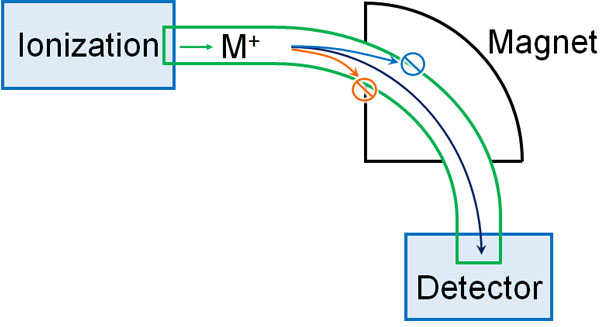By gradually changing the magnetic field and checking whether the ionized molecules are detected or not, it is possible to measure the molecular weight of the target compound.

The MS spectrum obtained in this way is called a mass spectrum. By looking at the spectral data observed by mass spectrometry, it is possible to infer what kind of substance the target compound is.

## In Many Cases, Electron Ionization Is Used in Mass Spectrometry

There are many different ways of doing these mass spectrometry methods. The following types are available.

• Electron Ionization (EI)
• Chemical Ionization (CI)
• Fast Atom Bombardment (FAB)
• Matrix Assisted Laser Desorption/Ionization (MALDI)
• Electrospray Ionization (ESI)

Unless you are a student studying for an exam, there is no point in understanding and remembering these detailed features. Therefore, we will not describe the detailed characteristics of each analytical method. In any case, it is enough to understand that there are several methods of mass spectrometry.

Of the MS methods, the most common method is electron ionization (EI). It is a method of measuring molecular weight by ionization through heating. The sample is ionized by bombarding it with electrons. Since only the target compound is measured, it is simple and data analysis is easy.

However, the electron ionization (EI) method cannot measure heat-sensitive compounds or high-molecular compounds such as proteins. In such cases, other analysis methods are used to measure molecular weight.

### Spectral Data and Readings in MS: Mass-to-Charge Ratio (m/z) and Charge (z)

When you measure a mass spectrum with MS, how do you read it? When you do mass spectrometry, you won’t detect a single peak. Mass spectra allow you to observe multiple peaks.

For example, when acetic acid is measured with MS, the data in the mass spectrum is as follows.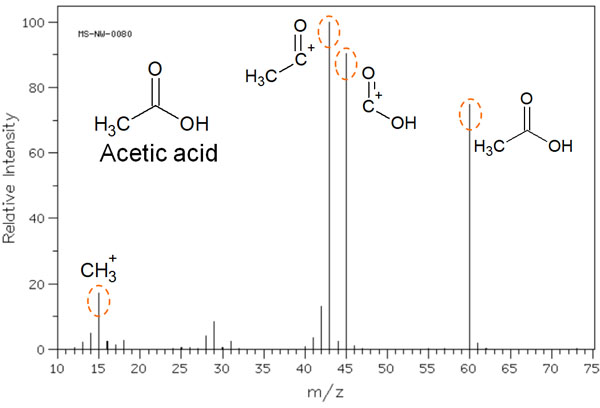As you can see, multiple peaks can be observed.

When a molecule is ionized, it is not necessarily ionized as acetic acid. Cleavage of the molecule results in the several molecule peaks shown in the figure above. As a result, these molecules are detected in the mass spectrum.

The horizontal axis is expressed as the mass-to-charge ratio (m/z). The m is the mass of the ion, and the z represents the charge. In short, let’s understand that m/z describes the molecular weight.

On the other hand, the vertical axis is ionic strength. The most common peak in the mass spectrum is 100%. In the previous figure, the molecule with the highest peak (m/z 43) has 100% ion intensity on the vertical axis. Compared to this intensity, the ratio of how much was detected in the mass spectrum is shown on the vertical axis.

We have used acetic acid as a case study to explain how to read a mass spectrum. Mass spectra produce not only the molecular weight of the target compound, but also several other molecules produced by hitting electrons; from this result, we can infer the structural formula.

### Molecules Are Split by Cleavage Reactions with Radical Cations

What kinds of molecules can be detected in the mass spectrum? Mass spectrometry uses a high-energy beam of electrons to eject electrons present in a molecule and create radical cations.

As the electrons fly away, unpaired electrons are created. If the positively charged radical cation is detected without causing any reactions, the weight of the target molecule can be measured.

However, radical cations are highly reactive. For example, with acetic acid, as noted earlier, multiple molecules can be detected.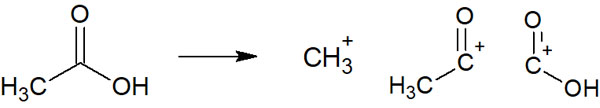In many cases, when a high-energy electron beam is applied by MS, the unshared electron pairs (electrons not involved in bonding) are forced out. For example, when nitrogen or oxygen atoms are present in the structural formula, the unshared electron pairs of these nitrogen or oxygen atoms tend to be ejected, producing radical cations.

For this reason, the presence of amino (NH2) and carbonyl (C=O) groups in the structural formula makes it easier to break the bond in that part of the molecule.

Other atoms such as chlorine, bromine, and iodine atoms bound to the benzene ring tend to fly away when they are hit by high-energy electrons.

Note that radical cleavage does not necessarily occur, and other reaction mechanisms can break the bonds between molecules. Sometimes the shape of the molecule can change dramatically due to a rearrangement reaction. The most common reaction, however, is that of radical cleavage. When radical cleavage occurs, multiple peaks are observed in the mass spectrum.

## Isotopes Such As Chlorine and Bromine are Detected

When making measurements with MS, the other thing to consider is the isotope. For example, the most common carbon atom is 12C. However, there is a small amount of 13C carbon. These isotopes have different masses. Therefore, they have different peaks that are detected by mass spectrometry.

13C is a small percentage. However, chlorine and bromine have a high percentage of isotopes. The ratio is as follows.

• 35Cl : 37Cl = 3 : 1
• 79Br : 81Br = 1 : 1

Therefore, if chlorine or bromine atoms are present in the structural formula, the isotope peaks will be clearly detected. For example, we have the following.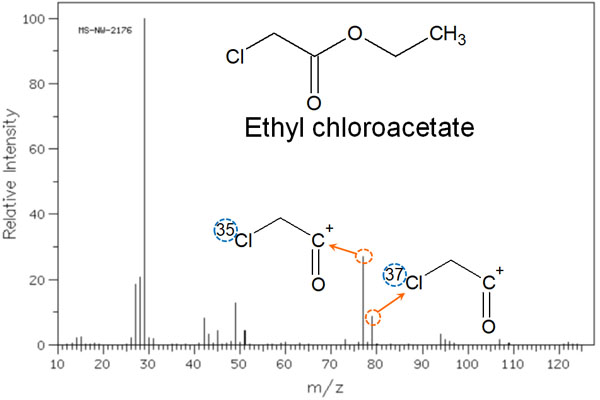In the case of chlorine atoms, the isotope ratio is 3:1, as described above. Therefore, the ionic strength on the vertical axis also shows a spectral peak at a ratio of 3:1.

### Isotope Peaks of Carbon Atoms Are Observed at M+1

As explained earlier, the probability of 13C’s existence is low compared to 12C. 13C is present at an existence ratio of 1.1%. So, can we ignore 13C when analyzing with MS? In mass spectra, it is not always sufficient to ignore 13C.

When the molecular weight is low, 13C is almost not observed because the ratio of 13C is only 1.1%. However, as the molecular weight increases and the number of carbon atoms increases, the 13C isotope peak becomes more and more visible. The original molecular weight is represented by M, and the 13C isotope peak is represented by M+1.

With n carbon atoms, the intensity ratio of M+ to [M+1]+ is as follows.

• M+ : [M+1]+ = 100 : 1.1 x n

The presence of n carbon atoms increases the probability of a 13C carbon atom being observed. For example, the following is a mass spectrum of 4-acetyl biphenyls.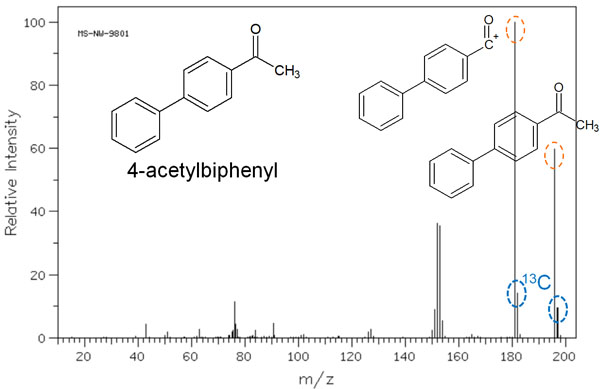The molecular weight of 4-acetyl biphenyl is 196. In the mass spectrum, you can see a smaller peak next to the main peak. This peak is meaningful, because 13C was observed as an isotope. This molecule has 14 carbon atoms, so a spectral peak of [M+1]+ is observed at 15.4% intensity.

• 1.1% × 14 = 15.4%

There are few opportunities to be aware of the isotopes when doing research. However, in mass spectra, we need to be strongly aware of the isotopes of carbon atoms.

## Mass Spectra Important in Structural Analysis

One of the most widely used analytical methods in many studies is mass spectrometry. It allows you to measure the molecular weight of a target compound and to infer what it contains as an unknown compound. It is a widely used analytical method in both organic chemistry and biochemistry.

It is not only the molecular weight that can be determined. Since radical cations are produced, intramolecular cleavage occurs, and other peaks can also be observed. From these peaks, it is possible to deduce the structural formula of the molecule.

The principle of this method is simple: the molecular weight is measured by the strength of the magnetic field. However, there are several analysis methods, and the electron ionization (EI) method, the most commonly used, has the disadvantage that it cannot measure molecules that decompose by heat. So, when doing MS, we have to choose the best method.

Furthermore, it is important to take isotopes into account. Understand these features and then use mass spectrometry in your scientific experiments.